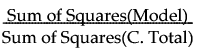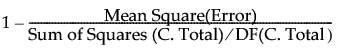Publication date: 07/30/2020

## Summary of Fit

The Summary of Fit report provides details such as RSquare calculations and the AICc and BIC values.

RSquare

Estimates the proportion of variation in the response that can be attributed to the model rather than to random error. Using quantities from the corresponding Analysis of Variance table, RSquare (also called the coefficient of multiple determination) is calculated as follows:An RSquare closer to 1 indicates a better fit to the data than does an RSquare closer to 0. An RSquare near 0 indicates that the model is not a much better predictor of the response than is the response mean.

Note: A low RSquare value suggests that there might be variables not in the model that account for the unexplained variation. However, if your data are subject to a large amount of inherent variation, even a useful regression model can have a low RSquare value. Read the literature in your research area to learn about typical RSquare values.

Adjusts the RSquare statistic for the number of parameters in the model. Rsquare Adj facilitates comparisons among models with different numbers of parameters. The computation uses the degrees of freedom. Using quantities from the corresponding Analysis of Variance table, RSquare Adj is calculated as follows:Root Mean Square Error

Estimates the standard deviation of the random error. This quantity is the square root of the Mean Square for Error in the Analysis of Variance report.

Note: Root Mean Square Error is commonly known as RMSE.

Mean of Response

Shows the overall mean of the response values.

Observations (or Sum Wgts)

Shows the number of observations used in the model.

This value is the same as the number of rows in the data table under the following conditions: there are no missing values, no excluded rows, and no column assigned to the role of Weight or Freq.

This value is the sum of the positive values in the Weight column if there is a column assigned to the role of Weight.

This value is the sum of the positive values in the Freq column if there is a column assigned to the role of Freq.

AICc

Shows the corrected Akaike Information Criterion value (AICc) and the Bayesian Information Criterion value (BIC). See Likelihood, AICc, and BIC in the Statistical Details section.

Note: AICc and BIC appear only if you have selected the AICc option from the Regression Reports menu or if you have set AICc as a Fit Least Squares preference.